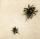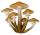# Real estate

Residential house has three entrances numbered by odd numbers arithmetic progression. The sum of the two numbers on the corner entrances is 50. Calculate the highest of this three numbers.

Result

m =  27

#### Solution:

m+(m-4)=50

2m = 54

m = 27

Calculated by our simple equation calculator.

Leave us a comment of example and its solution (i.e. if it is still somewhat unclear...):

Showing 0 comments:Be the first to comment!#### To solve this example are needed these knowledge from mathematics:

Do you solve Diofant problems and looking for a calculator of Diofant integer equations? Do you have a linear equation or system of equations and looking for its solution? Or do you have quadratic equation?

## Next similar examples:

1. Toy carsPavel has a collection of toy cars. He wanted to regroup them. But in the division of three, four, six, and eight, he was always one left. Only when he formed groups of seven, he divided everyone. How many toy cars have in the collection?
2. Divisible by 5How many three-digit odd numbers divisible by 5, which are in place ten's number 3?
3. FactoryIn the factory workers work in three shifts. In the first inning operates half of all employees in the second inning and a third in the third inning 200 employees. How many employees work at the factory?
4. RemainderA is an arbitrary integer that gives remainder 1 in the division with 6. B is an arbitrary integer that gives remainder 2 the division by. What makes remainder in division by 3 product of numbers A x B ?
5. Digit sumDetermine for how many integers greater than 900 and less than 1,001 has digit sum digit of the digit sum number 1.
6. Odd numbersThe sum of four consecutive odd numbers is 1048. Find those numbers ...
7. BasementsIn the first basement is more flies than the spiders, the second vice versa. Each basement had spiders and flies together 100 feet. Determine how many could be flies and spiders in the first and second basement. PS. We only need, when you write how many.
8. Solve 2Solve integer equation: a +b+c =30 a, b, c = can be odd natural number from this set (1,3,5,7,9,11,13,15)
9. Unknown numberIdentify unknown number which 1/5 is 40 greater than one tenth of that number.
10. Walnutsx walnuts were in the mission. Dano took 1/4 of nuts Michael took 1/8 from the rest and John took 34 nuts. It stayed here 29 nuts. Determine the original number of nuts.
11. Fifth of the numberThe fifth of the number is by 24 less than that number. What is the number?
12. EquationSolve the equation: 1/2-2/8 = 1/10; Write the result as a decimal number.
13. Mom and daughterMother is 39 years old. Her daughter is 15 years. For many years will mother be four times older than the daughter?
14. MushroomsFor five days, we have collected 410 mushrooms. Interestingly every day we have collected 10 mushrooms more than the preceding day. How many mushrooms we have collected during 4th day?
15. CandyPeter had a sachet of candy. He wanted to share with his friends. If he gave them 30 candies, he would have 62 candies. If he gave them 40 candies, he would miss 8 candies. How many friends did Peter have?
16. Apples 2How many apples were originally on the tree, if the first day fell one third, the second day quarter of the rest and on tree remained 45 apples?
17. Simple equationSolve for x: 3(x + 2) = x - 18